Download PresentationMemory 설계

# Memory 설계 - PowerPoint PPT Presentation

Memory 설계. Contents. Multiple drive Shifter Type declarations ROM(Read Only Memory) RAM(Random Access Memory) 실습내용. Multiple drive. 한 port 에 여러 개의 시그널 할당이 이루어 지는 경우 여러 개의 다른 신호가 할당되기 때문에 resolution function 에 따라 x 로 출력. architecture Behavioral of multi_drive isI am the owner, or an agent authorized to act on behalf of the owner, of the copyrighted work described.
Download Presentation## Memory 설계

An Image/Link below is provided (as is) to download presentation

Download Policy: Content on the Website is provided to you AS IS for your information and personal use and may not be sold / licensed / shared on other websites without getting consent from its author.While downloading, if for some reason you are not able to download a presentation, the publisher may have deleted the file from their server.

- - - - - - - - - - - - - - - - - - - - - - - - - - E N D - - - - - - - - - - - - - - - - - - - - - - - - - -
Presentation Transcript### Memory 설계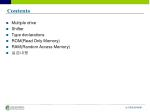Contents
• Multiple drive
• Shifter
• Type declarations
• ROM(Read Only Memory)
• RAM(Random Access Memory)
• 실습내용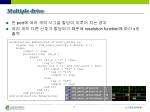Multiple drive
• 한 port에여러 개의 시그널 할당이 이루어 지는 경우
• 여러 개의 다른 신호가 할당되기 때문에 resolution function에 따라 x로 출력

architecture Behavioral of multi_drive is

signal reg : std_logic_vector( 1 downto 0 );

begin

output <= reg;

process( clk, rst_b )

begin

if( rst_b = '0' ) then

reg <= "00";

elsif( clk = '1' and clk'event ) then

if( input = "00" ) then

reg <= "11";

output <= reg;

else

reg <= "00";

output <= reg;

end if;

end if;

end process;

end architecture Behavioral;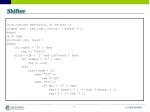Shifter

architecture Behavioral of shifter is

signal reg : std_logic_vector( 3 downto 0 );

begin

q <= reg;

process( clk, reset )

Begin

if( reset = '0' ) then

reg <= "0000";

elsif( clk = '1' and clk'event ) then

if( enable = '0' ) then

reg <= reg;

else

case( mode ) is

when "00" =>

reg <= pi;

when "01" =>

if( dir = '0' ) then

reg( 2 downto 0 ) <= reg( 3 downto 1 );

reg( 3 ) <= reg( 0 );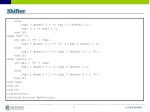Shifter

else

reg( 3 downto 1 ) <= reg ( 2 downto 0 );

reg( 0 ) <= reg( 3 );

end if;

when "10" =>

if( dir = '0' ) then

reg( 3 downto 0 ) <= '0' & reg( 3 downto 1 );

else

reg( 3 downto 0 ) <= reg( 2 downto 0 ) & '0';

end if;

when others =>

if( dir = '0' ) then

reg( 2 downto 0 ) <= reg( 3 downto 1 );

else

reg( 3 downto 0 ) <= reg( 2 downto 0 ) & '0';

end if;

end case;

end if;

end if;

end process;

end architecture Behavioral;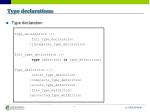Type declarations
• Type declaration

Type_declaration ::=

full_type_declaration

|incomplete_type_declaration

Full_type_declaration ::=

type identifier is type_definition;

Type_definition ::=

scalar_type_definition

|composite_type_definition

|access_type_definition

|file_type_definition

|protected_type_definition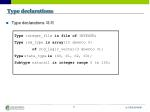Type declarations
• Type declarations 예제

Typeinteger_fileis file of INTEGER;

Type rom_type is array(15 downto 0)

of std_logic_vector(3 downto 0);

Typestate_typeis (S0, S1, S2, S3);

Subtype natural1 is integer range 0 to 150;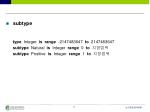subtype

type Integer is range -2147483647 to 2147483647

subtype Natural is Integer range 0 to지정범위

subtype Positive is Integer range 1 to지정범위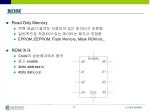ROM
• Read Only Memory
• 전원 공급이 끊겨도 저장되어 있는 데이터가 보존됨
• 일반적으로 저장되어 있는 데이터는 제조시 저장됨
• EPROM, EEPROM, Flash Memory, Mask ROM etc..
• ROM 예제
• Clock의상승에지에서 동작
• 동기 enable
• 4bits address in
• 4bits data outROM
• Module

entity rominfr is

port( clk, en : instd_logic;

addr : instd_logic_vector( 3 downto 0 );

data : outstd_logic_vector( 3 downto 0 ) );

end entity rominfr;

architecture Behavioral of rominfr is

typerom_typeisarray(15 downto 0) ofstd_logic_vector(3 downto 0);

constant ROM : rom_type :=

( "0001", "0010", "0011", "0100", "0101", "0110", "0111", "1000"

, "1001", "1010", "1011", "1100", "1101", "1110", "1111", "0000"

);

begin

process( clk )

begin

if( clk = '1' and clk'event ) then

if( en = '1' ) then

data <= ROM( conv_integer( addr ) );

end if;

end if;

end process;

end architecture Behavioral;ROM
• Simulation resultRAM
• Random Access Memory
• 읽기와 쓰기가 가능함
• 전원 공급 차단시 저장되어 있는 데이터 손실
• SRAM, DRAM etc..
• RAM 예제
• Clock의 상승에지에서 동작
• 동기 enable
• 동기 writeenable
• 4bits Data input
• 4bits Data output

4

4

4RAM
• 진리표RAM
• 실습내용
• Enable을 가지는 single-port RAM 설계
• 앞 페이지의 RAM 예제를 충족시킬 것(enable이 write enable보다 우선)
• 주어진 entity 및 testbench를 사용할 것
• Clock 주기 : 10 ns
• Testbench input 초기값 0
• entity

entity raminfr is

port( clk, en, we : instd_logic;

addr, di : instd_logic_vector( 3 downto 0 );

do : outstd_logic_vector( 3 downto 0 ) );

end entity raminfr;RAM
• Testbench

wait for 53 ns;

di <= "1111“;

we <= '1';

wait for 10 ns;

en <= '1';

wait for 10 ns;

di <= "1110";

wait for 10 ns;

di <= "1101";

wait for 10 ns;

di <= "1100";

wait for 10 ns;

di <= "1011";

wait for 10 ns;

di <= "0011";

wait for 10 ns;

di <= "0000";

wait for 10 ns;

en <= '0';

di <= "0010";

wait for 10 ns;

we <= '0';

wait for 10 ns;

wait for 10 ns;

en <= '1';

wait for 10 ns;

wait for 10 ns;

wait for 10 ns;

wait for 10 ns;

report "Simulation end";

wait;RAM
• Simulation resultRAM 설계 참고

type ram_type is array (15 downto 0) of std_logic_vector( 3 downto 0);

signal ram : ram_type;

signal read_addr : std_logic_vector (3 downto 0);